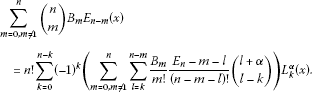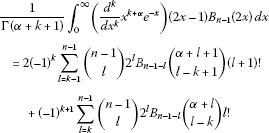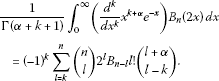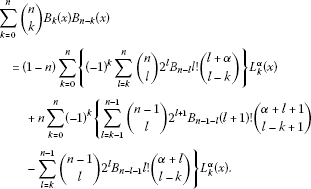Theory and Modern Applications

# Some identities on Bernoulli and Euler polynomials arising from the orthogonality of Laguerre polynomials

## Abstract

In this paper, we derive some interesting identities on Bernoulli and Euler polynomials by using the orthogonal property of Laguerre polynomials.

## 1 Introduction

The generalized Laguerre polynomials are defined by

(1.1)

From (1.1), we note that

${L}_{n}^{\alpha }\left(x\right)=\sum _{r=0}^{n}\frac{{\left(-1\right)}^{r}\left(\genfrac{}{}{0}{}{n+\alpha }{n-r}\right){x}^{r}}{r!}\phantom{\rule{1em}{0ex}}\left(\text{see [1–3]}\right).$
(1.2)

By (1.2), we see that ${L}_{n}^{\alpha }\left(x\right)$ is a polynomial with degree n. It is well known that Rodrigues’ formula for ${L}_{n}^{\alpha }\left(x\right)$ is given by

${L}_{n}^{\alpha }\left(x\right)=\frac{{x}^{-\alpha }{e}^{x}}{n!}\left(\frac{{d}^{n}}{d{x}^{n}}\left({e}^{-x}{x}^{n+\alpha }\right)\right)\phantom{\rule{1em}{0ex}}\left(\text{see [1–3]}\right).$
(1.3)

From (1.3) and a part of integration, we note that

${\int }_{0}^{\mathrm{\infty }}{x}^{\alpha }{e}^{-x}{L}_{m}^{\alpha }\left(x\right){L}_{n}^{\alpha }\left(x\right)\phantom{\rule{0.2em}{0ex}}dx=\frac{\mathrm{\Gamma }\left(\alpha +n+1\right)}{n!}{\delta }_{m,n},$
(1.4)

where ${\delta }_{m,n}$ is a Kronecker symbol. As is well known, Bernoulli polynomials are defined by the generating function to be

$\frac{t}{{e}^{t}-1}{e}^{xt}={e}^{B\left(x\right)t}=\sum _{n=0}^{\mathrm{\infty }}{B}_{n}\left(x\right)\frac{{t}^{n}}{n!}\phantom{\rule{1em}{0ex}}\left(\text{see [1–29]}\right),$
(1.5)

with the usual convention about replacing ${B}^{n}\left(x\right)$ by ${B}_{n}\left(x\right)$.

In the special case, $x=0$, ${B}_{n}\left(0\right)={B}_{n}$ are called the n th Bernoulli numbers. By (1.5), we get

${B}_{n}\left(x\right)=\sum _{l=0}^{n}\left(\genfrac{}{}{0}{}{n}{l}\right){B}_{n-l}{x}^{l}\phantom{\rule{1em}{0ex}}\left(\text{see [1–29]}\right).$
(1.6)

The Euler numbers are defined by

${E}_{0}=1,\phantom{\rule{2em}{0ex}}{\left(E+1\right)}^{n}+{E}_{n}=2{\delta }_{0,n}\phantom{\rule{1em}{0ex}}\left(\text{see [27, 28]}\right),$
(1.7)

with the usual convention about replacing ${E}^{n}$ by ${E}_{n}$.

In the viewpoint of (1.6), the Euler polynomials are also defined by

${E}_{n}\left(x\right)={\left(E+x\right)}^{n}=\sum _{l=0}^{n}\left(\genfrac{}{}{0}{}{n}{l}\right){E}_{n-l}{x}^{l}\phantom{\rule{1em}{0ex}}\left(\text{see [11–24]}\right).$
(1.8)

From (1.7) and (1.8), we note that the generating function of the Euler polynomial is given by

$\frac{2}{{e}^{t}+1}{e}^{xt}={e}^{E\left(x\right)t}=\sum _{n=0}^{\mathrm{\infty }}{E}_{n}\left(x\right)\frac{{t}^{n}}{n!}\phantom{\rule{1em}{0ex}}\left(\text{see [15–29]}\right).$
(1.9)

By (1.5) and (1.9), we get

$\frac{2}{{e}^{t}+1}{e}^{xt}=\frac{1}{t}\left(2-2\frac{2}{{e}^{t}+1}\right)\left(\frac{t{e}^{xt}}{{e}^{t}-1}\right)=-2\sum _{n=0}^{\mathrm{\infty }}\left(\sum _{l=0}^{n}\frac{{E}_{l+1}}{l+1}\left(\genfrac{}{}{0}{}{n}{l}\right){B}_{n-l}\left(x\right)\right)\frac{{t}^{n}}{n!}.$
(1.10)

Thus, by (1.10), we see that

${E}_{n}\left(x\right)=-2\sum _{l=0}^{n}\left(\genfrac{}{}{0}{}{n}{l}\right)\frac{{E}_{l+1}}{l+1}{B}_{n-l}\left(x\right).$
(1.11)

By (1.7) and (1.8), we easily get

$\frac{t}{{e}^{t}-1}{e}^{xt}=\frac{t}{2}\left(\frac{2{e}^{xt}}{{e}^{t}+1}\right)+\left(\frac{t}{{e}^{t}-1}\right)\left(\frac{2{e}^{x}t}{{e}^{t}+1}\right).$
(1.12)

Thus, by (1.12), we see that

${B}_{n}\left(x\right)=\sum _{k=0,k\ne 1}^{n}\left(\genfrac{}{}{0}{}{n}{k}\right){B}_{k}{E}_{n-k}\left(x\right).$
(1.13)

Throughout this paper, we assume that $\alpha \in \mathbb{Q}$ with $\alpha >-1$. Let ${\mathbb{P}}_{n}=\left\{p\left(x\right)\in \mathbb{Q}\left[x\right]|degp\left(x\right)\le n\right\}$ be the inner product space with the inner product

$〈p\left(x\right),q\left(x\right)〉={\int }_{0}^{\mathrm{\infty }}{x}^{\alpha }{e}^{-x}p\left(x\right)q\left(x\right)\phantom{\rule{0.2em}{0ex}}dx,$

where $p\left(x\right),q\left(x\right)\in {\mathbb{P}}_{n}$. From (1.4), we note that $\left\{{L}_{0}^{\alpha }\left(x\right),{L}_{1}^{\alpha }\left(x\right),\dots ,{L}_{n}^{\alpha }\left(x\right)\right\}$ is an orthogonal basis for ${\mathbb{P}}_{n}$.

In this paper, we give some interesting identities on Bernoulli and Euler polynomials which can be derived by an orthogonal basis $\left\{{L}_{0}^{\alpha }\left(x\right),{L}_{1}^{\alpha }\left(x\right),\dots ,{L}_{n}^{\alpha }\left(x\right)\right\}$ for ${\mathbb{P}}_{n}$.

## 2 Some identities on Bernoulli and Euler polynomials

Let $p\left(x\right)\in {\mathbb{P}}_{n}$. Then $p\left(x\right)$ can be generated by $\left\{{L}_{0}^{\alpha }\left(x\right),{L}_{1}^{\alpha }\left(x\right),\dots ,{L}_{n}^{\alpha }\left(x\right)\right\}$ in ${\mathbb{P}}_{n}$ to be

$p\left(x\right)=\sum _{k=0}^{n}{C}_{k}{L}_{k}^{\alpha }\left(x\right),$
(2.1)

where

$\begin{array}{rcl}〈p\left(x\right),{L}_{k}^{\alpha }\left(x\right)〉& =& {C}_{k}〈{L}_{k}^{\alpha }\left(x\right),{L}_{k}^{\alpha }\left(x\right)〉\\ =& {C}_{k}{\int }_{0}^{\mathrm{\infty }}{x}^{\alpha }{e}^{-x}{L}_{k}^{\alpha }\left(x\right){L}_{k}^{\alpha }\left(x\right)\phantom{\rule{0.2em}{0ex}}dx\\ =& {C}_{k}\frac{\mathrm{\Gamma }\left(\alpha +k+1\right)}{k!}.\end{array}$
(2.2)

From (2.2), we note that

$\begin{array}{rcl}{C}_{k}& =& \frac{k!}{\mathrm{\Gamma }\left(\alpha +k+1\right)}〈p\left(x\right),{L}_{k}^{\alpha }\left(x\right)〉\\ =& \frac{k!}{\mathrm{\Gamma }\left(\alpha +k+1\right)}\frac{1}{k!}{\int }_{0}^{\mathrm{\infty }}\left(\frac{{d}^{k}}{d{x}^{k}}{x}^{k+\alpha }{e}^{-x}\right)p\left(x\right)\phantom{\rule{0.2em}{0ex}}dx\\ =& \frac{1}{\mathrm{\Gamma }\left(\alpha +k+1\right)}{\int }_{0}^{\mathrm{\infty }}\left(\frac{{d}^{k}}{d{x}^{k}}{x}^{k+\alpha }{e}^{-x}\right)p\left(x\right)\phantom{\rule{0.2em}{0ex}}dx.\end{array}$
(2.3)

Let us take $p\left(x\right)={\sum }_{m=0,m\ne 1}^{n}\left(\genfrac{}{}{0}{}{n}{m}\right){B}_{m}{E}_{n}-m\left(x\right)\in {\mathbb{P}}_{n}$. Then, from (2.3), we have

$\begin{array}{rcl}{C}_{k}& =& \frac{1}{\mathrm{\Gamma }\left(\alpha +k+1\right)}{\int }_{0}^{\mathrm{\infty }}\left(\frac{{d}^{k}}{d{x}^{k}}{x}^{k+\alpha }{e}^{-x}\right)\sum _{m=0,m\ne 1}^{n}\left(\genfrac{}{}{0}{}{n}{m}\right){B}_{n}{E}_{n-m}\left(x\right)\phantom{\rule{0.2em}{0ex}}dx\\ =& \frac{{\left(-1\right)}^{k}}{\mathrm{\Gamma }\left(\alpha +k+1\right)}\sum _{m=0,m\ne 1}^{n-k}\sum _{l=k}^{n-m}\left(\genfrac{}{}{0}{}{n}{m}\right)\left(\genfrac{}{}{0}{}{n-m}{l}\right){B}_{m}{E}_{n-m-l}\frac{l!}{\left(l-k\right)!}{\int }_{0}^{\mathrm{\infty }}{x}^{l+\alpha }{e}^{-x}\phantom{\rule{0.2em}{0ex}}dx\\ =& \frac{{\left(-1\right)}^{k}}{\mathrm{\Gamma }\left(\alpha +k+1\right)}\sum _{m=0,m\ne 1}^{n-k}\sum _{l=k}^{n-m}\left(\genfrac{}{}{0}{}{n}{m}\right)\left(\genfrac{}{}{0}{}{n-m}{l}\right){B}_{m}{E}_{n-m-l}\frac{l!}{\left(l-k\right)!}\mathrm{\Gamma }\left(l+\alpha +1\right)\\ =& {\left(-1\right)}^{k}\sum _{m=0,m\ne 1}^{n-k}\sum _{l=k}^{n-m}\left(\genfrac{}{}{0}{}{n}{m}\right)\left(\genfrac{}{}{0}{}{n-m}{l}\right){B}_{m}{E}_{n-m-l}\frac{l!}{\left(l-k\right)!}\frac{\left(l+\alpha \right)\left(l+\alpha -1\right)\cdots \alpha }{\left(\alpha +k\right)\left(\alpha +k-1\right)\cdots \alpha }\\ =& {\left(-1\right)}^{k}n!\sum _{m=0,m\ne 1}^{n-k}\sum _{l=k}^{n-m}\frac{{B}_{m}}{m!}\frac{{E}_{n-m-l}}{\left(n-m-l\right)!}\left(\genfrac{}{}{0}{}{l+\alpha }{l-k}\right).\end{array}$
(2.4)

Therefore, by (2.1) and (2.4), we obtain the following theorem.

Theorem 2.1 For $n\in {\mathbb{Z}}_{+}$, we haveFrom (1.13), we can derive the following corollary.

Corollary 2.2 For $n\in {\mathbb{Z}}_{+}$, we have

${B}_{n}\left(x\right)=n!\sum _{k=0}^{n}{\left(-1\right)}^{k}\left(\sum _{m=0,m\ne 1}^{n-k}\sum _{l=k}^{n-m}\frac{{B}_{m}}{m!}\frac{{E}_{n}-m-l}{\left(n-m-l\right)!}\left(\genfrac{}{}{0}{}{l+\alpha }{l-k}\right)\right){L}_{k}^{\alpha }\left(x\right).$

Let us take $p\left(x\right)={\sum }_{l=0}^{n}\left(\genfrac{}{}{0}{}{n}{l}\right)\frac{{E}_{l+1}}{l+1}{B}_{n-l}\left(x\right)$. By the same method, we get

$\begin{array}{rcl}{C}_{k}& =& \frac{1}{\mathrm{\Gamma }\left(\alpha +k+1\right)}{\int }_{0}^{\mathrm{\infty }}\left(\frac{{d}^{k}}{d{x}^{k}}{x}^{k+\alpha }{e}^{-x}\right)\sum _{l=0}^{n}\left(\genfrac{}{}{0}{}{n}{l}\right)\frac{{E}_{l+1}}{l+1}{B}_{n-l}\left(x\right)\phantom{\rule{0.2em}{0ex}}dx\\ =& \frac{1}{\mathrm{\Gamma }\left(\alpha +k+1\right)}\sum _{l=0}^{n-k}\sum _{m=0}^{n-l}\left(\genfrac{}{}{0}{}{n}{l}\right)\left(\genfrac{}{}{0}{}{n-l}{m}\right)\frac{{E}_{l+1}}{l+1}{B}_{n-l-m}{\int }_{0}^{\mathrm{\infty }}\left(\frac{{d}^{k}}{d{x}^{k}}{x}^{k+\alpha }{e}^{-x}\right){x}^{m}\phantom{\rule{0.2em}{0ex}}dx\\ =& \frac{{\left(-1\right)}^{k}}{\mathrm{\Gamma }\left(\alpha +k+1\right)}\sum _{l=0}^{n-k}\sum _{m=k}^{n-l}\left(\genfrac{}{}{0}{}{n}{l}\right)\left(\genfrac{}{}{0}{}{n-l}{m}\right)\frac{{E}_{l+1}}{l+1}{B}_{n-l-m}\frac{m!}{\left(m-k\right)!}\mathrm{\Gamma }\left(m+\alpha +1\right)\\ =& {\left(-1\right)}^{k}\sum _{l=0}^{n-k}\sum _{m=k}^{n-l}\left(\genfrac{}{}{0}{}{n}{l}\right)\left(\genfrac{}{}{0}{}{n-l}{m}\right)\frac{m!}{\left(m-k\right)!}\frac{{E}_{l+1}}{\left(l+1\right)}{B}_{n-l-m}\frac{\left(\alpha +m\right)\left(\alpha +m-1\right)\cdots \alpha }{\left(\alpha +k\right)\left(\alpha +k-1\right)\cdots \alpha }\\ =& {\left(-1\right)}^{k}n!\sum _{l=0}^{n-k}\sum _{m=k}^{n-l}\left(\genfrac{}{}{0}{}{\alpha +m}{m-k}\right)\frac{{E}_{l+1}}{\left(l+1\right)!}\frac{{B}_{n-l-m}}{\left(n-l-m\right)!}.\end{array}$
(2.5)

Therefore, by (1.11), (2.1), and (2.5), we obtain the following theorem.

Theorem 2.3 For $n\in {\mathbb{Z}}_{+}$, we have

$-\frac{{E}_{n}\left(x\right)}{2}=n!\sum _{k=0}^{n}{\left(-1\right)}^{k}\left(\sum _{l=0}^{n-k}\sum _{m=k}^{n-l}\left(\genfrac{}{}{0}{}{\alpha +m}{m-k}\right)\frac{{E}_{m+1}}{\left(m+1\right)!}\frac{{B}_{n-m-l}}{\left(n-m-l\right)!}\right){L}_{k}^{\alpha }\left(x\right).$

For $n\in \mathbb{N}$ with $n\ge 2$ and $m\in {\mathbb{Z}}_{+}$ with $n-m\ge 0$, we have

$\begin{array}{rcl}{B}_{n-m}\left(x\right){B}_{m}\left(x\right)& =& \sum _{r}\left\{\left(\genfrac{}{}{0}{}{n-m}{2r}\right)m+\left(\genfrac{}{}{0}{}{m}{2r}\right)\left(n-m\right)\right\}\frac{{B}_{2r}{B}_{n-2r}\left(x\right)}{n-2r}\\ +{\left(-1\right)}^{m+1}\frac{\left(n-m\right)!m!}{n!}{B}_{n}\in {\mathbb{P}}_{n}\phantom{\rule{1em}{0ex}}\left(\text{see }\right).\end{array}$
(2.6)

Let us take $p\left(x\right)={B}_{n-m}\left(x\right){B}_{m}\left(x\right)\in {\mathbb{P}}_{n}$. Then $p\left(x\right)$ can be generated by an orthogonal basis $\left\{{L}_{0}^{\alpha }\left(x\right),{L}_{1}^{\alpha }\left(x\right),\dots ,{L}_{n}^{\alpha }\left(x\right)\right\}$ in ${\mathbb{P}}_{n}$ to be

$p\left(x\right)=\sum _{k=0}^{n}{C}_{k}{L}_{k}^{\alpha }\left(x\right).$
(2.7)

From (2.3), (2.6), and (2.7), we note that

$\begin{array}{rcl}{C}_{k}& =& \frac{1}{\mathrm{\Gamma }\left(\alpha +k+1\right)}{\int }_{0}^{\mathrm{\infty }}\left(\frac{{d}^{k}}{d{x}^{k}}{x}^{k+\alpha }{e}^{-x}\right)p\left(x\right)\phantom{\rule{0.2em}{0ex}}dx\\ =& \frac{1}{\mathrm{\Gamma }\left(\alpha +k+1\right)}\sum _{r=0}^{\left[\frac{n}{2}\right]}\left\{\left(\genfrac{}{}{0}{}{n-m}{2r}\right)m+\left(\genfrac{}{}{0}{}{m}{2r}\right)\left(n-m\right)\right\}\\ ×\frac{{B}_{2r}}{n-2r}{\int }_{0}^{\mathrm{\infty }}\left(\frac{{d}^{k}}{d{x}^{k}}{x}^{k+\alpha }{e}^{-x}\right){B}_{n-2r}\left(x\right)\phantom{\rule{0.2em}{0ex}}dx\\ =& \frac{1}{\mathrm{\Gamma }\left(\alpha +k+1\right)}\sum _{r=0}^{\left[\frac{n}{2}\right]}\left\{\left(\genfrac{}{}{0}{}{n-m}{2r}\right)m+\left(\genfrac{}{}{0}{}{m}{2r}\right)\left(n-m\right)\right\}\frac{{B}_{2r}}{n-2r}\\ ×\sum _{l=0}^{n-2r}\left(\genfrac{}{}{0}{}{n-2r}{l}\right){B}_{n-2r-l}{\int }_{0}^{\mathrm{\infty }}\left(\frac{{d}^{k}}{d{x}^{k}}{x}^{k+\alpha }{e}^{-x}\right){x}^{l}\phantom{\rule{0.2em}{0ex}}dx\\ =& \frac{1}{\mathrm{\Gamma }\left(\alpha +k+1\right)}\sum _{r=0}^{\left[\frac{n}{2}\right]}\sum _{l=0}^{n-2r}\left\{\left(\genfrac{}{}{0}{}{n-m}{2r}\right)m+\left(\genfrac{}{}{0}{}{m}{2r}\right)\left(n-m\right)\right\}\left(\genfrac{}{}{0}{}{n-2r}{l}\right)\\ ×\frac{{B}_{2r}{B}_{n-2r-l}}{n-2r}{\int }_{0}^{\mathrm{\infty }}\left(\frac{{d}^{k}}{d{x}^{k}}{x}^{k+\alpha }{e}^{-x}\right){x}^{l}\phantom{\rule{0.2em}{0ex}}dx\\ =& \frac{{\left(-1\right)}^{k}}{\mathrm{\Gamma }\left(\alpha +k+1\right)}\sum _{r=0}^{\left[\frac{n-k}{2}\right]}\sum _{l=k}^{n-2r}\left\{\left(\genfrac{}{}{0}{}{n-m}{2r}\right)m+\left(\genfrac{}{}{0}{}{m}{2r}\right)\left(n-m\right)\right\}\left(\genfrac{}{}{0}{}{n-2r}{l}\right)\\ ×\frac{{B}_{2r}{B}_{n-2r-l}l!}{\left(n-2r\right)\left(l-k\right)!}\mathrm{\Gamma }\left(\alpha +l+1\right).\end{array}$
(2.8)

It is easy to show that

$\begin{array}{rcl}\frac{\mathrm{\Gamma }\left(\alpha +l+1\right)}{\mathrm{\Gamma }\left(\alpha +k+1\right)\left(l-k\right)!}& =& \frac{\left(\alpha +l\right)\left(\alpha +l-1\right)\cdots \alpha \mathrm{\Gamma }\left(\alpha \right)}{\left(\alpha +k\right)\left(\alpha +k-1\right)\cdots \alpha \mathrm{\Gamma }\left(\alpha \right)\left(l-k\right)!}\\ =& \frac{\left(\alpha +l\right)\left(\alpha +l-1\right)\cdots \left(\alpha +k+1\right)}{\left(\alpha -k\right)!}=\left(\genfrac{}{}{0}{}{\alpha +l}{l-k}\right).\end{array}$
(2.9)

By (2.8) and (2.9), we get

$\begin{array}{rcl}{C}_{k}& =& {\left(-1\right)}^{k}\sum _{r=0}^{\left[\frac{n-k}{2}\right]}\sum _{l=k}^{n-2r}\left\{\left(\genfrac{}{}{0}{}{n-m}{2r}\right)m+\left(\genfrac{}{}{0}{}{m}{2r}\right)\left(n-m\right)\right\}\\ ×\left(\genfrac{}{}{0}{}{n-2r}{l}\right)\left(\genfrac{}{}{0}{}{\alpha +l}{l-k}\right)\frac{l!{B}_{2r}{B}_{n-2r-l}}{\left(n-2r\right)}.\end{array}$
(2.10)

Therefore, by (2.7) and (2.10), we obtain the following theorem.

Theorem 2.4 For $n\in \mathbb{N}$ with $n\ge 2$ and $m\in {\mathbb{Z}}_{+}$ with $n-m\ge 0$, we have

$\begin{array}{rcl}{B}_{n-m}\left(x\right){B}_{m}\left(x\right)& =& \sum _{k=0}^{n}{\left(-1\right)}^{k}\left\{\sum _{r=0}^{\left[\frac{n-k}{2}\right]}\sum _{l=k}^{n-2r}\left(\left(\genfrac{}{}{0}{}{n-m}{2r}\right)m+\left(\genfrac{}{}{0}{}{m}{2r}\right)\left(n-m\right)\right)\\ ×\left(\genfrac{}{}{0}{}{n-2r}{l}\right)\left(\genfrac{}{}{0}{}{\alpha +l}{l-k}\right)\frac{l!{B}_{2r}{B}_{n-2r-l}}{\left(n-2r\right)}\right\}{L}_{k}^{\alpha }\left(x\right).\end{array}$

It is easy to show that

$\frac{{t}^{2}{e}^{t\left(x+y\right)}}{{\left({e}^{t}-1\right)}^{2}}=\left(x+y-1\right)\frac{{t}^{2}{e}^{t\left(x+y-1\right)}}{{e}^{t}-1}-\frac{{t}^{2}\phantom{\rule{0.2em}{0ex}}d}{dt}\left(\frac{{e}^{t\left(x+y-1\right)}}{{e}^{t}-1}\right).$
(2.11)

From (2.11), we have

$\sum _{k=0}^{n}\left(\genfrac{}{}{0}{}{n}{k}\right){B}_{k}\left(x\right){B}_{n-k}\left(y\right)=\left(1-n\right){B}_{n}\left(x+y\right)+\left(x+y-1\right)n{B}_{n-1}\left(x+y\right)\phantom{\rule{1em}{0ex}}\left(\text{see }\right).$
(2.12)

Let $x=y$. Then by (2.12), we get

$\sum _{k=0}^{n}\left(\genfrac{}{}{0}{}{n}{k}\right){B}_{k}\left(x\right){B}_{n-k}\left(x\right)=\left(1-n\right){B}_{n}\left(2x\right)+\left(2x-1\right){B}_{n-1}\left(2x\right).$
(2.13)

Let us take $p\left(x\right)={\sum }_{k=0}^{n}\left(\genfrac{}{}{0}{}{n}{k}\right){B}_{k}\left(x\right){B}_{n-k}\left(x\right)\in {\mathbb{P}}_{n}$. Then $p\left(x\right)$ can be generated by an orthogonal basis $\left\{{L}_{0}^{\alpha }\left(x\right),{L}_{1}^{\alpha }\left(x\right),\dots ,{L}_{n}^{\alpha }\left(x\right)\right\}$ in ${\mathbb{P}}_{n}$ to be

$p\left(x\right)=\sum _{k=0}^{n}\left(\genfrac{}{}{0}{}{n}{k}\right){B}_{k}\left(x\right){B}_{n-k}\left(x\right)=\sum _{k=0}^{n}{C}_{k}{L}_{k}^{\alpha }\left(x\right).$
(2.14)

From (2.3), (2.13), and (2.14), we can determine the coefficients ${C}_{k}$’s to be

$\begin{array}{rcl}{C}_{k}& =& \frac{1}{\mathrm{\Gamma }\left(\alpha +k+1\right)}{\int }_{0}^{\mathrm{\infty }}\left(\frac{{d}^{k}}{d{x}^{k}}{x}^{k+\alpha }{e}^{-x}\right)p\left(x\right)\phantom{\rule{0.2em}{0ex}}dx\\ =& \frac{1}{\mathrm{\Gamma }\left(\alpha +k+1\right)}\left\{\left(1-n\right){\int }_{0}^{\mathrm{\infty }}\left(\frac{{d}^{k}}{d{x}^{k}}{x}^{k+\alpha }{e}^{-x}\right){B}_{n}\left(2x\right)\phantom{\rule{0.2em}{0ex}}dx\\ +n{\int }_{0}^{\mathrm{\infty }}\left(\frac{{d}^{k}}{d{x}^{k}}{x}^{k+\alpha }{e}^{-x}\right)\left(2x-1\right){B}_{n-1}\left(2x\right)\phantom{\rule{0.2em}{0ex}}dx\right\}.\end{array}$
(2.15)

By simple calculation, we get(2.16)

and(2.17)

Therefore, by (2.13), (2.14), (2.15), (2.16), and (2.17), we obtain the following theorem.

Theorem 2.5 For $n\in {\mathbb{Z}}_{+}$, we get## References

1. Carlitz L: An integral for the product of two Laguerre polynomials. Boll. Unione Mat. Ital. 1962, 17(17):25–28.

2. Carlitz L: On the product of two Laguerre polynomials. J. Lond. Math. Soc. 1961, 36: 399–402. 10.1112/jlms/s1-36.1.399

3. Cangul N, Kurt V, Ozden H, Simsek Y: On the higher-order w - q -Genocchi numbers. Adv. Stud. Contemp. Math. 2009, 19(1):39–57.

4. Akemann G, Kieburg M, Phillips MJ: Skew-orthogonal Laguerre polynomials for chiral real asymmetric random matrices. J. Phys. A 2010., 43(37): Article ID 375207

5. Bayad A, Kim T: Identities for the Bernoulli, the Euler and the Genocchi numbers and polynomials. Adv. Stud. Contemp. Math. 2010, 20(2):247–253.

6. Bayad A: Modular properties of elliptic Bernoulli and Euler functions. Adv. Stud. Contemp. Math. 2010, 20(3):389–401.

7. Carlitz L: Note on the integral of the product of several Bernoulli polynomials. J. Lond. Math. Soc. 1959, 34: 361–363. 10.1112/jlms/s1-34.3.361

8. Carlitz L: Some generating functions for Laguerre polynomials. Duke Math. J. 1968, 35: 825–827. 10.1215/S0012-7094-68-03587-4

9. Costin RD: Orthogonality of Jacobi and Laguerre polynomials for general parameters via the Hadamard finite part. J. Approx. Theory 2010, 162(1):141–152. 10.1016/j.jat.2009.04.002

10. Ding D, Yang J: Some identities related to the Apostol-Euler and Apostol-Bernoulli polynomials. Adv. Stud. Contemp. Math. 2010, 20(1):7–21.

11. Hansen ER: A Table of Series and Products. Prentice Hall, Englewood Cliffs; 1975.

12. Kudo A: A congruence of generalized Bernoulli number for the character of the first kind. Adv. Stud. Contemp. Math. 2000, 2: 1–8.

13. Kim T, Choi J, Kim YH, Ryoo CS: On q -Bernstein and q -Hermite polynomials. Proc. Jangjeon Math. Soc. 2011, 14(2):215–221.

14. Kim T: A note on q -Bernstein polynomials. Russ. J. Math. Phys. 2011, 18(1):73–82. 10.1134/S1061920811010080

15. Kim T: Some identities on the q -Euler polynomials of higher order and q -Stirling numbers by the fermionic p -adic integral on ${\mathbb{Z}}_{p}$ . Russ. J. Math. Phys. 2009, 16(4):484–491. 10.1134/S1061920809040037

16. Kim T: Symmetry of power sum polynomials and multivariate fermionic p -adic invariant integral on ${\mathbb{Z}}_{p}$ . Russ. J. Math. Phys. 2009, 16(1):93–96. 10.1134/S1061920809010063

17. Ozden H, Cangul IN, Simsek Y: Multivariate interpolation functions of higher-order q -Euler numbers and their applications. Abstr. Appl. Anal. 2008., 2008: Article ID 390857

18. Choi J, Kim DS, Kim T, Kim YH: Some arithmetic identities on Bernoulli and Euler numbers arising from the p -adic integrals on ${\mathbb{Z}}_{p}$ . Adv. Stud. Contemp. Math. 2012, 22(2):239–247.

19. Ozden H, Cangul IN, Simsek Y: Remarks on q -Bernoulli numbers associated with Daehee numbers. Adv. Stud. Contemp. Math. 2009, 18(1):41–48.

20. Rim SH, Lee SJ: Some identities on the twisted $\left(h,q\right)$ -Genocchi numbers and polynomials associated with q -Bernstein polynomials. Int. J. Math. Math. Sci. 2011., 2011: Article ID 482840

21. Rim SH, Bayad A, Moon EJ, Jin JH, Lee SJ: A new construction on the q -Bernoulli polynomials. Adv. Differ. Equ. 2011., 2011: Article ID 34

22. Rim SH, Jin JH, Moon EJ, Lee SJ: Some identities on the q -Genocchi polynomials of higher-order and q -Stirling numbers by the fermionic p -adic integral on ${\mathbb{Z}}_{p}$ . Int. J. Math. Math. Sci. 2010., 2010: Article ID 860280

23. Ryoo CS: On the generalized Barnes type multiple q -Euler polynomials twisted by ramified roots of unity. Proc. Jangjeon Math. Soc. 2010, 13(2):255–263.

24. Ryoo CS: A note on the Frobenius-Euler polynomials. Proc. Jangjeon Math. Soc. 2011, 14(4):495–501.

25. Ryoo CS: Some relations between twisted q -Euler numbers and Bernstein polynomials. Adv. Stud. Contemp. Math. 2011, 21(2):217–223.

26. Ryoo CS: Some identities of the twisted q -Euler numbers and polynomials associated with q -Bernstein polynomials. Proc. Jangjeon Math. Soc. 2011, 14(2):239–248.

27. Simsek Y: Generating functions of the twisted Bernoulli numbers and polynomials associated with their interpolation functions. Adv. Stud. Contemp. Math. (Kyungshang) 2008, 16(2):251–278.

28. Simsek Y: Special functions related to Dedekind-type DC-sums and their applications. Russ. J. Math. Phys. 2010, 17(4):495–508. 10.1134/S1061920810040114

29. Simsek Y: On p -adic twisted q - L -functions related to generalized twisted Bernoulli numbers. Russ. J. Math. Phys. 2006, 13(3):340–348. 10.1134/S1061920806030095

## Acknowledgements

The authors would like to express their deep gratitude to the referees for their valuable suggestions and comments.

## Author information

Authors

### Corresponding author

Correspondence to Taekyun Kim.

### Competing interests

The authors declare that they have no competing interests.

### Authors’ contributions

All authors contributed equally to the manuscript and typed, read, and approved the final manuscript.

## Authors’ original submitted files for images

Below are the links to the authors’ original submitted files for images.

## Rights and permissions

Reprints and Permissions

Kim, T., Rim, SH., Dolgy, D. et al. Some identities on Bernoulli and Euler polynomials arising from the orthogonality of Laguerre polynomials. Adv Differ Equ 2012, 201 (2012). https://doi.org/10.1186/1687-1847-2012-201

• Accepted:

• Published:

• DOI: https://doi.org/10.1186/1687-1847-2012-201

### Keywords

• Differential Equation
• Generate Function
• Partial Differential Equation
• Ordinary Differential Equation
• Functional Analysis